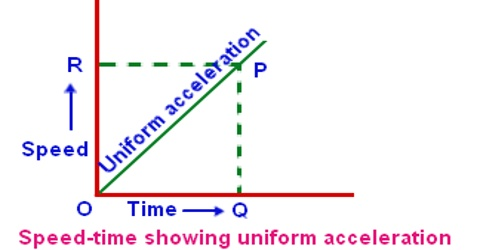# Uniform Acceleration related to Motion

Uniform acceleration: If the acceleration always remains constant, then that acceleration is called uniform acceleration.

The acceleration of a freely falling body due to the action of gravitation is uniform acceleration. Equal force acts on a body of uniform acceleration. Both the magnitude and direction of uniform acceleration remain constant. So, uniform acceleration occurs when the speed of an object changes at a constant rate.

In figure (a), uniform acceleration is shown by successive change of velocity with time along a straight line. In figure (b) uniform acceleration has been shown by a graph. Here the value of uniform acceleration is 2 ms-2. In case of uniform acceleration the graph is a straight line and the slope becomes constant.The uniform acceleration of a body is 10 ms-2 means that the velocity of the body changes in each second by 10 ms-1 in the same direction.

Example:

A car was travelling at a speed of 19.4 m/s, the driver saw a rabbit on the road and slammed on the breaks. After 6.0 seconds the car came to a halt, how far did the car travel from the point where the brakes were first pressed to the point where the car stopped?

We are given:

Vi = 19.4 m/s and Vf = 0 km/h

t = 6s and d = ?

Our formula for distance is d = 1/2 ( Vf + Vi) × t

d = 1/2 (19.4 + 0) × 6

d = 58.332

The car stopped after 58 metres.